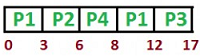# GATE Solved Paper 2017-19 - GATE 2017 Shift 1

>>>>>>>>GATE 2017 Shift 1

• A

4• B

3• C

5• D

6• Option : A
• Explanation :
The regular expression can be described as “All strings over {a, b} ending with “ba” or “bb”. The minimal DFA accepting L is having 4 states:• A

0.05• B

0.25• C

0.50• D

0.02• Option : A
• Explanation :
Number of memory access in 1000 instructions = 1.4 x 1000
= 1,400
∴ Miss rate = 7/(1400 x 0.1) = 0.05

 Process Arrival time Burst time P1 0 7 P2 3 3 P3 5 5 P4 6 2
If the pre-emptive shortest remaining time first scheduling algorithm is used to schedule the processes, then the average waiting time across all processes is _______ milliseconds.

Note: Numerical Type question.

• A

1• B

2• C

3• D

4• Option : C
• Explanation :Turn Around Time
P1 = 12-0 = 12
P2 = 6-3 = 3
P3 = 17-5 = 12
P4 = 8 - 6 = 2
Waiting Time
P1 = 12-7 = 5
P2 = 3-3 = 0
P3 = 12-5 = 7
P4 = 2 - 2 = 0
Average Waiting time = (7+0+5+0)/4 = 3.0
Therefore, option C is correct

 EMP EmpId Emp Name Dept Name 1. XYA AA 2. XYB AA 3. XYC AA 4. XYD AA 5. XYE AB 6. XYF AB 7. XYG AB 8. XYH AC 9. XYI AC 10. XYJ AC 11. XYK AD 12. XYL AD 13. XYM AE
 SELECT AVG (EC.Num)FROM ECWHERE(DeptName, Num)IN (SELECT DeptName, COUNT(EmpId)AS     EC(DeptName,Num) FROMEMP GROUP BY DeptName)
The output of executing the SQL query is _____.

Note – Numerical Type question

• A

2.6• B

2.3• C

3.2• D

3.6• Option : A
• Explanation :  EC Dept Name Num AA 4 AB 3 AC 3 AD 2 AE 1
Avg(NUM) = 13/5 = 2.6

• A

10• B

9• C

18• D

20• Option : C
• Explanation :
Given, v= Total vertices = 10
e = v – 1 =9
Degree = 2 * e = 18
Therefore, option C is correct
Related Quiz.
GATE 2017 Shift 1